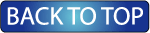Oceanographic Observations, Hudson Shelf Valley, U.S. Geological Survey Open-File Report 02-217

## Principal Axes of Currents

Principal axes for both the hour-averaged and low-passed currents were computed for the entire record and by month. Major and minor axes, orientation, and ellipticity were computed from the east (u) and north (v) current components as:

major axis = [0.5 (UU + VV) + R] / n}(1/2)
minor axis = [0 5 (UU + VV) - R] / n}(1/2)
orientation = 90° - 0.5 tan-1 [2 UV / (UU - VV)]
ellipticity = 1 - (minor axis / major axis)

where

UV = Sum(u*v) - n*U*V

UU = Sum(u*u) - n*U*U
VV= Sum(v*v) - n*V*V
R = [ (0.5 (UU - VV) )2 + (UV)2 ](1/2)

and U and V are the means of the east and north velocity components, respectively. Sum means sum over the entire data set of n valves. The orientation is measured clockwise from true north. 0° is true north, and 90° is east.

#### Principal Axes for Entire Record (ADCP/MAVS/BASS Observations)

 Key major = major axis minor = minor axis orient = orientation (measured clockwise from north) ellip = ellipticity alh = hour-averaged data lp = low-passed data

### Principal Axes for Each Station

 Site 5 mbs 15 mbs 30 mbs 45 mbs Bottom A No Data B C D No Data No Data E No Data No Data F[an error occurred while processing this directive]